# Normal line – Concave lens

Before we go any further, you need to understand what the "normal line" is in connection with concave lenses.

There are several definitions of the normal line of a concave lens.

Normal line is the line perpendicular (at 90°) to the tangent line.

Or

The normal line is an imaginary line at 90° to where the ray touches the surface.

NOTE: For explanation of tangent line see Mammoth Memory

But at Mammoth Memory we believe that you should think of a bow held by a Norman warrier in which the bow is the curve and the arrow is the normal line.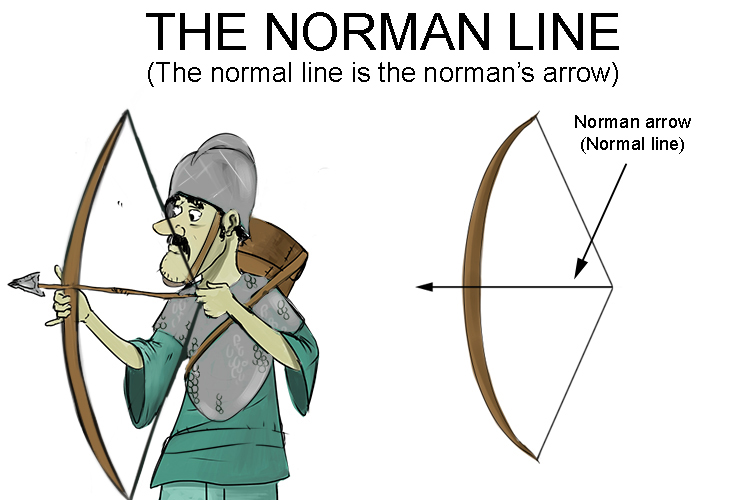The Norman's arrow is the line perpendicular (at 90°) to the tangent line of the bow. The arrow is the imaginary line at 90° to the bow.

So, at the point where the ray touches the surface of the concave lens, draw a tangent to the surface.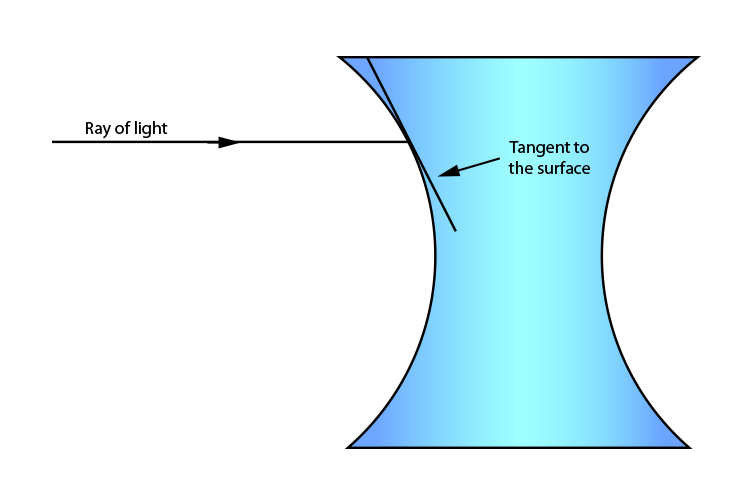Then draw a perpendicular line (at 90°) to the tangent.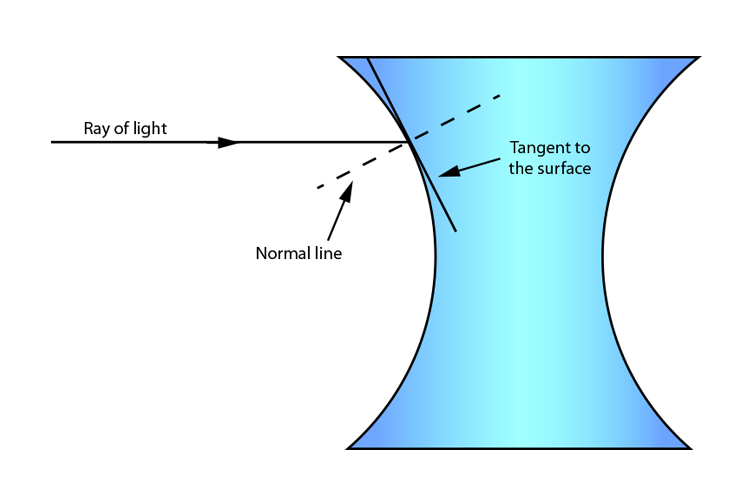The normal line is the arrow that would exist if the curve were the bow.

Then you can remove the tangent line.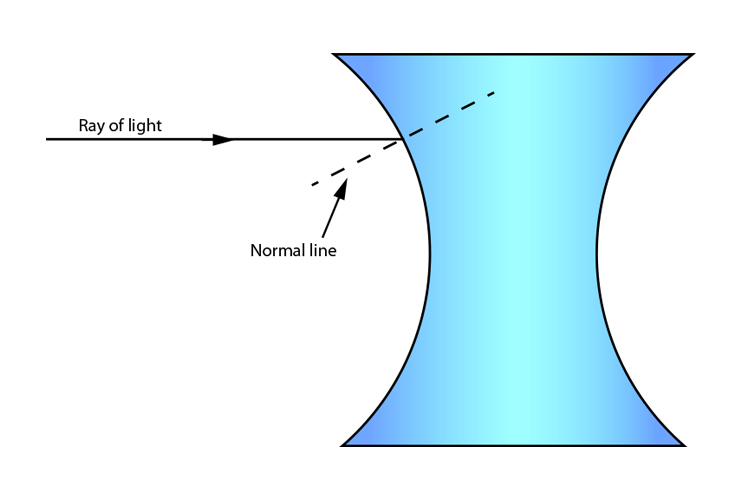This is the normal line and it is perpendicular to the concave lens's surface drawn at the point of contact.

The ray of light will now refract (change direction) at the surface of the concave lens. For the rules of refraction and how much the ray of light bends, see Mammoth Memory refraction.

Because at this level of physics we are only dealing with thin lenses, the actual amount the light refracts (changes direction) across the lens is minimal. As long as the ray of light is shown to turn slightly towards the normal line on the diagram this will be sufficient for exam purposes.

NOTE: Most of the diagrams we show exaggerate the width of the lens for ease of explanation.

Once the ray of light is inside the lens the rules of refraction again apply as the ray travels through the lens and approaches the exit surface. At the point where the ray touches the exit surface, draw a tangent to the outer surface: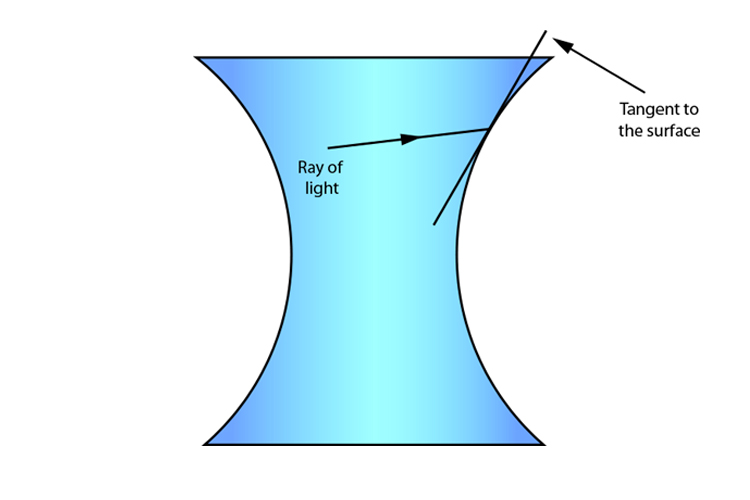Then draw a perpendicular (at 90°) to the tangent to find the normal line.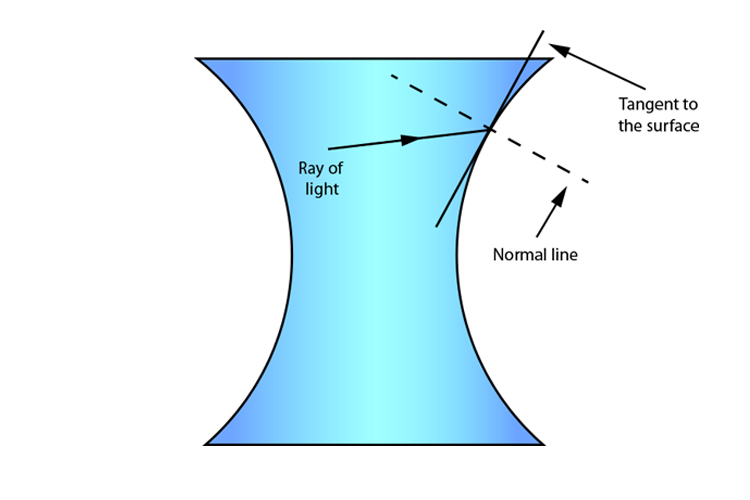Then you can remove the tangent.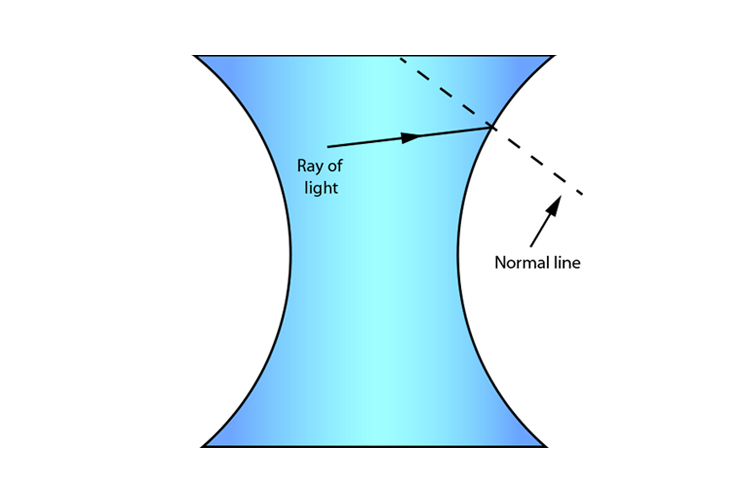This is the other normal line (there are two for each ray of light, one as it enters and another as it exits the lens) and it is perpendicular to the concave lens surface drawn at the point of contact.